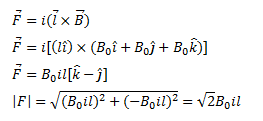# A wire of length I carries a current i along the X-axis.

Question:

A wire of length I carries a current i along the X-axis. A magnetic field exists which is given as $\vec{B}=B_{0}(\hat{\imath}+\hat{\jmath}+\hat{k})_{\mathrm{T}}$. Find the magnitude of the magnetic force acting on the wire.

Solution: